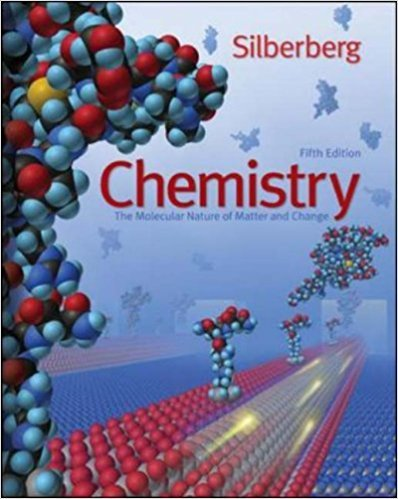×
×

# Calculate each of the following quantities: (a) Volume ofISBN: 9780077216504 103

## Solution for problem 3.101 Chapter 3

Chemistry: The Molecular Nature of Matter and Change | 5th Edition

• Textbook Solutions
• 2901 Step-by-step solutions solved by professors and subject experts
• Get 24/7 help from StudySoup virtual teaching assistantsChemistry: The Molecular Nature of Matter and Change | 5th Edition

4 5 0 283 Reviews
19
5
Problem 3.101

Calculate each of the following quantities: (a) Volume of 2.050 M copper(II) nitrate that must be diluted with water to prepare 750.0 mL of a 0.8543 M solution (b) Volume of 1.63 M calcium chloride that must be diluted with water to prepare 350. mL of a 2.86 10 2 M chloride ion solution (c) Final volume of a 0.0700 M solution prepared by diluting 18.0 mL of 0.155 M lithium carbonate with water

Step-by-Step Solution:
Step 1 of 3

MGT 218: Chapter 6: Continuous Random Variables and the Normal distribution (PART 2) with Solution for Exercises on Text book. Theory : Standardizing a Normal Distribution : Converting an x Value to a z Value : For a normal random variable x, a particular value of x can be converted to its corresponding z value by using the formula : where Mean and standard deviation are the mean and...

Step 2 of 3

Step 3 of 3

##### ISBN: 9780077216504

Unlock Textbook Solution